# Practical Astronomy

## practical astronomy

[′prak·ti·kəl ə′strän·ə·mē]
(astronomy)
That part of astronomy concerned with the use of information acquired by an observer in the solution of problems determining latitude and longitude on sea or land and directions on the earth's surface by the help of celestial objects.
McGraw-Hill Dictionary of Scientific & Technical Terms, 6E, Copyright © 2003 by The McGraw-Hill Companies, Inc.
The following article is from The Great Soviet Encyclopedia (1979). It might be outdated or ideologically biased.

## Practical Astronomy

a branch of astrometry dealing with the study of astronomical instruments and the methods of determining time, geographic coordinates, and azimuths from astronomical observations. It is divided into geodetic astronomy, nautical astronomy, and aviation astronomy, depending on the conditions under which its activities are performed. The methods used are based on the rules of spherical astronomy and the use of star catalogs, the compilation of which is a task of fundamental astrometry. Practical astronomy arose in early antiquity to meet the economic needs of society.

The instruments used in practical astronomy measure angles in the horizontal and vertical planes and determine the times at which heavenly bodies cross vertical circles and almucantars. They include the theodolite, the zenith telescope, the vertical circle, the portable transit instrument, the photographic zenith tube, and marine and aircraft sextants. Quartz-crystal clocks and marine chronometers are used to measure time, and a radio time-signal receiver is used to determine longitudes.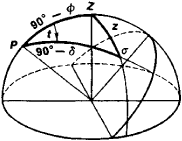Figure 1

Practical astronomy uses several methods to determine the local times—which is equivalent to determining the clock correction u —the latitude ϕ, the longitude λ, and the azimuth A to an object on the earth. In the methods discussed below, a is the azimuth, ζ is the zenith distance, α is the right ascension, δ is the declination, t is the hour angle of a celestial body, s is the local time, and T is the clock reading at the moment of observation.

(1) Determination of u and ϕ from measurements of the zenith distance ζ of a heavenly body σ. It follows from the parallactic triangle PZσ (Figure 1), where P is the celestial pole, Z is the zenith, and σ is the place of the heavenly body, that

(1) cos Z = sin ϕ sin δ + cos ϕ cos δ cos t

where

(2) t = T + u - α

First, the values of α and δ for the observed body are obtained from an astronomical catalog, and the body’s zenith distance ζ at the moment T is measured. Equations (1) and (2) can then be used to calculate the clock correction u if ϕ is known, or to calculate ϕ if u is known. If neither u nor ϕ is known, then equations (1) and (2) are solved by the method of successive approximations. An alternate method requires that two stars, one near the meridian and the other near the prime vertical, be observed. The two systems of equations (1) and (2) obtained can then be solved simultaneously. When the two stars are at culmination, the following equations are valid:

(3) ϕ = δs + Zs and ϕ = δN — ZN

Here the subscripts N and S denote the heavenly body at culmination north and south of the zenith. Since ζ cannot be exactly measured on the meridian, it is measured near the meridian, and a necessary correction is introduced into the calculations.

(2) Determination of u and ϕ from observations of pairs of stars at equal zenith distances z. In 1874 the Russian geodesist N. Ia. Tsinger proposed a method for determining u from observations of the times at which two stars cross the same almucantar (the Tsinger method). The stars are observed near the prime vertical, one star to the east of it and the other to the west; they are symmetric about the meridian. A similar method of determining ϕ from observations of a pair of stars at equal zenith distances near the meridian was proposed in 1887 by the Russian explorer M.V. Pevtsov (seePEVTSOV METHOD). In both methods the observations are simple to perform and the results are highly accurate.

(3) Simultaneous determination of u and ϕ. The Soviet scientist V.V. Kavraiskii, in the period 1924–36, and A. V. Mazaev, in the period 1943–45, proposed methods of determining u and ϕ simultaneously (seeKAVRAISKII METHOD and MAZAEV’S METHOD). In the Kavraiskii method two pairs of stars are observed, each pair having equal zenith distances z, while in Mazaev’s method a series of stars is observed at the almucantar with z = 45° or z = 30°.

(4) Determination of ϕ by the Talcott method. This method, proposed in 1857 by the American geodesist A. Talcott, is based on the measurement of a small difference in zenith distance between two stars at culmination on different sides of the zenith. Taking the half-sum of both equations in (3) yields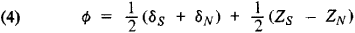The stars are selected so that the difference between their zenith distances is not greater than the diameter of the instrument’s effective field of view, that is, at most 10″-15″, and the difference between their right ascensions is 5–20 min (in observations of both stars at their upper culminations). During observations, the zenith telescope or theodolite is adjusted to the average zenith distance of the pair of stars at 0° azimuth in order to observe the star that is at culmination south of the zenith; the tube is adjusted to a 180° azimuth to observe the star at culmination north of the zenith. The quantity zs — ZN is measured with an eyepiece micrometer. The Talcott method has become widespread, particularly at international stations that study the motion of the terrestrial poles.

(5) Determination of u and ϕ from observations with a photographic zenith tube. A number of time service and latitude service observatories determine u and ϕ from combined observations with photographic zenith tubes. The image of a star is fixed on a photographic plate moving at the same velocity as the star. The moments of time are marked on the plate. Stars are observed within a narrow zone around the zenith, bounded by the tube’s effective field of view. The axis of the instrument is fixed toward the zenith; this is controlled using a mercury horizon.

(6) Determination of u by means of a transit instrument. This method has become widespread in time services and for high-precision determinations of longitude. The transit times of a series of stars as they cross the meridian are observed and recorded either with a contact micrometer or with photomultipliers. Corrections are calculated by the formula

(5) u = α - T

A similar method using a theodolite was proposed by the Russian geodesist N. D. Pavlov in 1912. In some cases, u is determined by observing the passage of a star across the vertical circle of Polaris (the Dellen method).

(7) Determination of λ. The east longitude of the place of observation is related to the universal time S and the local time s by the relationship

(6) λ = s- S = T + u- S

where u is determined by one of the above methods and S is determined from time signals relayed by several radio stations and received over the course of 24 hours.

(8) Determination of A. The most widespread method of de-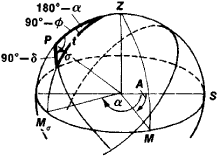Figure 2

termining A uses a theodolite to measure the horizontal angle between the bearing Mσ of Polaris (Figure 2) and the bearing M of an object on the earth. This is followed by a calculation of the azimuth of Polaris at the moment of observation 5. The equation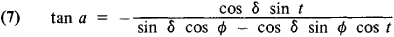where t = s — α, is used for this purpose. The azimuth A of the object is found from the equation

(8) A = a + M - Mσ

In geodesy the azimuth is often determined by observing the moments at which stars with a high ζ (50°-70°) pass near the meridian.

(9) Determination of ϕ and λ by means of celestial lines of position, proposed by the American seaman T. Sumner in 1843. In nautical and aviation astronomy, and λ must be determined rapidly, but less accuracy is required; the method of celestial lines of position, the essence of which is clear from Figure 3, is therefore generally used. From the point m, whose geographic coordinates are to be determined, the zenith distance z1 of a celestial body σ1 (with coordinates α and δι) is measured, and the geographic coordinates of the projection Σι of the heavenly body onto the earth’s surface—the stellar point—are determined from the formulas ϕι = δ and λι = α1S (east longitude). The circle of radius z1 with center at Σι passes along the globe through the point m. Once the value of z2 for a second star has been measured, a second circle with radius z2 and center at Σ2 is drawn; the desired point m is at one of the two points of intersection of these circles. It is not difficult to choose the correct point, since the approximate location of the observation position is often known. In actual practice, a map is used in place of a globe; one draws segments of curves on the map corresponding to the arcs of the circles near the point where the circles intersect. These segments are called celestial lines of position or Sumner lines.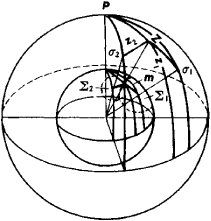Figure 3

All the problems that arise in practical astronomy are of great importance for astronomy, geodesy, and geophysics. Determination of ϕ, λ, and A is necessary for the orientation of triangulation networks used as an aid in cartographic work and in the study of the figure of the earth. Periodic and secular motions of the terrestrial poles have been established from studies of the variability of ϕ. Redetermination of the longitudes of observatories over a period of time has provided data necessary for investigating continental drift.

### REFERENCES

Blazhko, S. N. Kurs prakticheskoi astronomii, 3rd ed. Moscow-Leningrad, 1951.
Belobrov, A. P. Morekhodnaia astronomiia. Leningrad, 1954.
Vorob’ev, L. M. Astronomicheskaia navigatsiia letatel’nykh apparatov. Moscow, 1968.

V. P. SHCHEGLOV

References in periodicals archive ?
"The weather was closing in, with increasing cloud, so instead of the planned practical astronomy class, I gave the students an introductory demonstration of how to use the CCD camera on one of the observatory's automated 0.35-meter telescopes," Dr.
Alexander Wilson born; the first professor of practical astronomy at Glasgow; in 1769 November he observed a very large sunspot and proposed that sunspots are cavities in the luminous matter surrounding the Sun.
The research question is What types of changes occur in teachers' self-efficacy when they participate in practical astronomy research and specialist professional learning opportunities?
First, the realization that observatories are required to adumbrate current astronomical theory led to major advancement in practical astronomy through the construction of observatories, with the first two in Baghdad and Damascus and then at Maragha (13th century) and Samarqand (15th century).
It's more or less an update of his 2001 book of nearly the same name, but this edition in Patrick Moore's Practical Astronomy Series actually costs less.
Work that was not possible to carry out in Denmark but possible in the States was multifarious: close cooperation between theoretical and observational astronomy; spectral photometry requiring comparisons between theory and practical astronomy; scientific confirmations and refinement of both theory and experiment; and practical work in geometrical optics.
Though overly technical for a work intended for a more general audience, the author usefully explores the parallel traditions of theoretical and practical astronomy among astronomers in fourteenth-century Maragaha and fifteenth-century Samarqand.
Subsequently, the agendas and expectations of a consummate Royal Society man clashed with those Flamsteed hoped to secure for practical astronomy.
The most engaging practical astronomy activities are best delivered with sustained support from the astronomical community, and this community needs to know how to support them.
A new entry in Patrick Moore's Practical Astronomy Series, Field Guide to Meteors and Meteorites is encyclopedic in scope yet organized in a way that allows you to dip in with just a toe or to dive in headfirst.
John was a generous and kind man, always willing to give advice and help in practical astronomy matters.
David Arditti's book is the fourth volume in Patrick Moore's Practical Astronomy series that spotlights how to construct telescope enclosures.

Site: Follow: Share:
Open / Close